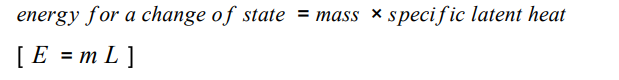# AQQ GCSE Physics Changes of state and specific latent heat

1. What is the energy needed for a substance to change its state called?
2. Do five star jumps.
3. When a change of state occurs, the energy supplied changes the energy stored (internal energy). What is not changed.
4. Sketch a heating curve for water. Temperature against energy supplied.
5. Define the specific latent heat of a substance.
6. Look at this equation.7. What is the unit for mass?
8. What is the unit for specific latent heat?
9. What is the unit of energy for a change of state?
10. Rearrange the equation so that m = and L =
11. What does the L refer to?
12. Define the specific latent heat of fusion.
13. Define the specific latent of vaporisation.
14. Spend twenty minutes on your Nintendo switch.
15. Sketch a heating curve and a cooling curve and label where the changes of state occur.
16. Why are the regions on the graph where there is a change of state flat?
17. What is the difference between specific heat capacity and specific latent heat?